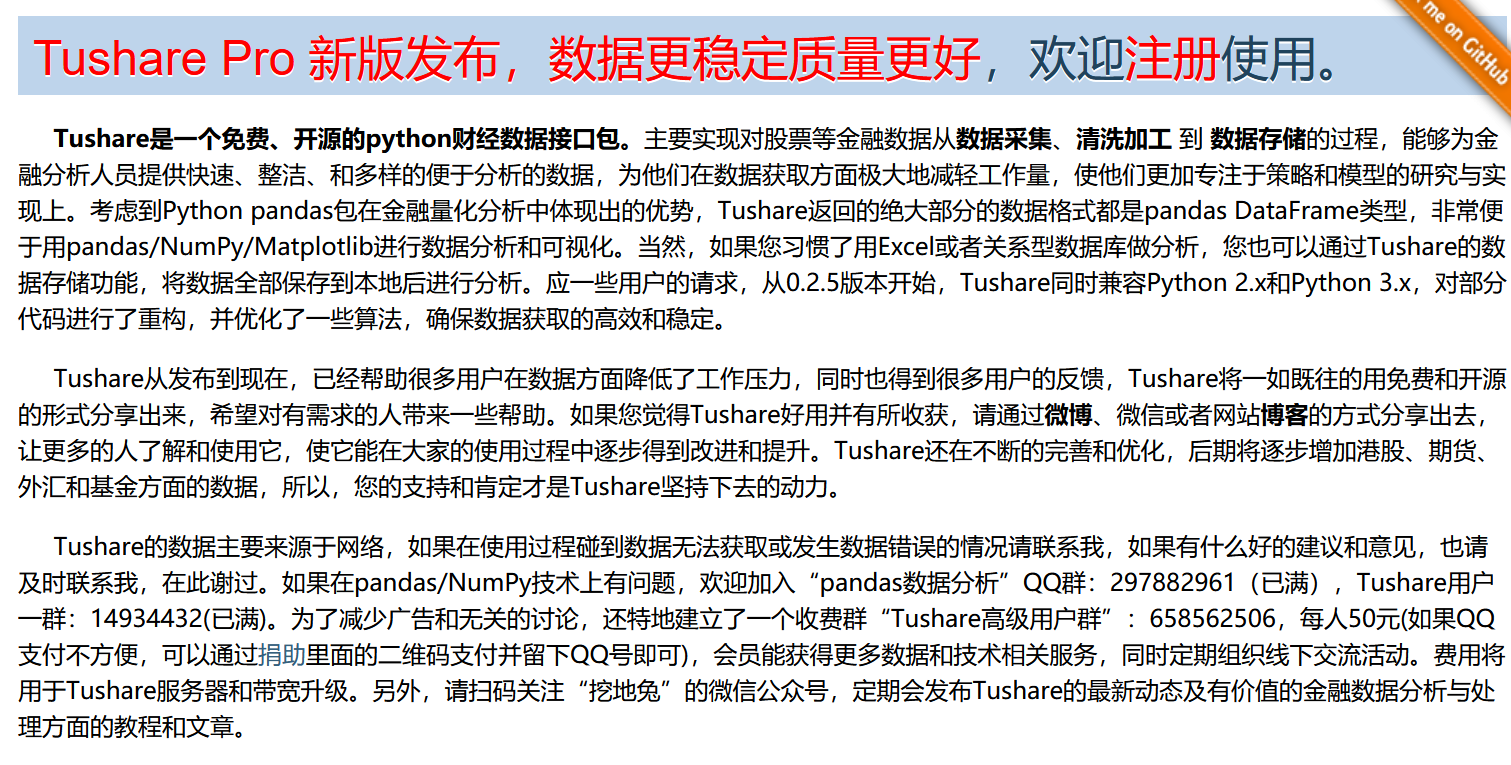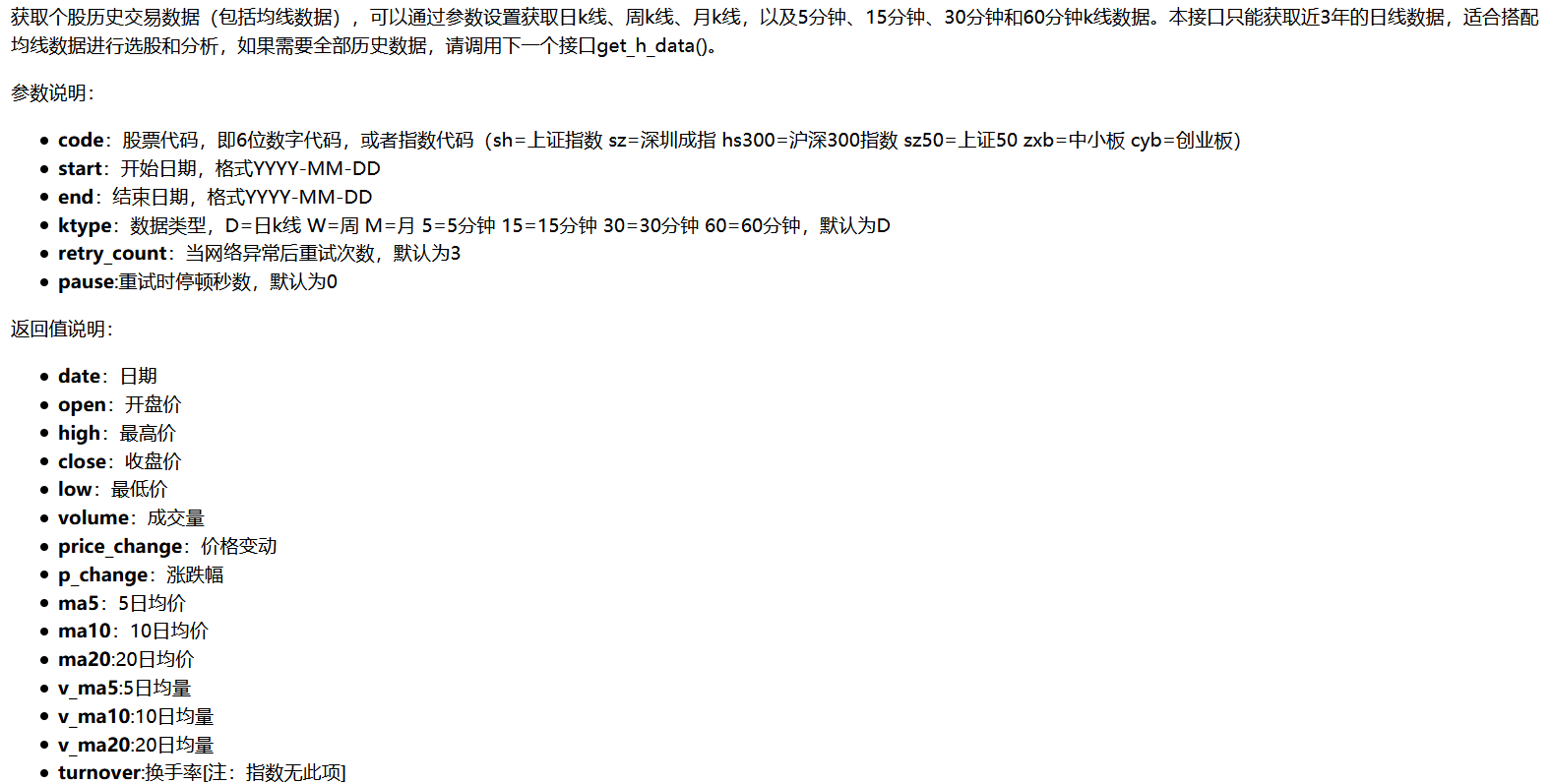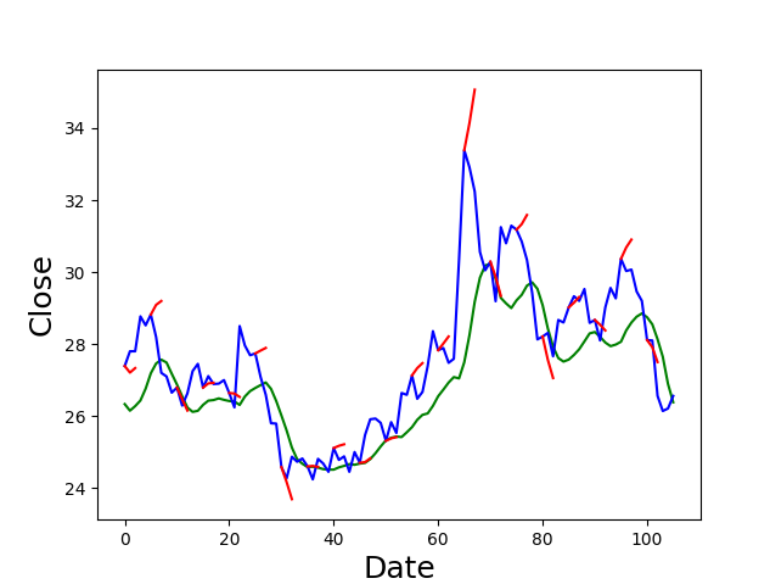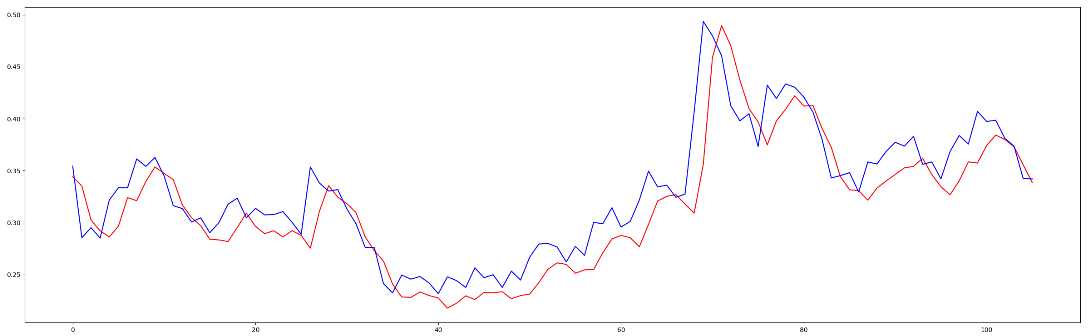• 里面使用Keras搭建了了LSTM模型进行股价预测，详细介绍了模型的搭建、调试、评估等过程，在数据处理上包含了滑窗、归一化等步骤，是一份非常详细的代码。
• 使用LSTM预测股价案例，超级精简，便于理解，是LSTM入门的好案例。
• 机器学习实战项目教你股价预测，实战项目，机器学习实战项目教你股价预测
• 基于金融高频数据与互联网资讯的股价预测研究.pdf
• 关于股价预测常见的股价预测方法机器学习预测股价功能快捷键合理的创建标题，有助于目录的生成如何改变文本的样式插入链接与图片如何插入一段漂亮的代码片生成一个适合你的列表创建一个表格设定内容居中、居左、居右...


关于股价预测
常见的股价预测方法机器学习预测股价特征选择

常见的股价预测方法
目前常见的股价预测方法已经十分完善了，但是大多数还是研究性质的，实用价值较少。原因是大部分预测股价的其实都是通过前一天的股价来预测第二天的股价，而没有利用更多的信息。记录一下常见的股价预测的方法。为了简化将股价预测转换为二分类问题，即预测第二天股价的信息。
机器学习预测股价
机器学习主要是通过以下几种算法，实现也十分简单，通过调取sklearn的库，几行代码即可完成。 1. 多元线性回归算法
start = time.time()
clf = LinearRegression()
clf.fit(train_data,train_label)
end1 = time.time()
print('********************训练处理时间：**********',end1-start)
print('**************训练结束，开始预测******************************')
pre = clf.predict(dev_data)
score = log_loss(dev_label,clf.predict(dev_data))
evaluate(model_type,pre,dev_label)
end2 = time.time()
print('********************测试处理时间：**********', end2 - end1)

2.K-means算法
 start = time.time()
clf  = KNeighborsRegressor(n_neighbors=8)
clf.fit(train_data,train_label)
end1 = time.time()
print('********************训练处理时间：**********', end1 - start)
print('**************训练结束，开始预测******************************')
pre = clf.predict(dev_data)
evaluate(model_type,pre,dev_label)
end2 = time.time()
print('********************测试处理时间：**********', end2 - end1)

3. 决策树
 start = time.time()
clf = DecisionTreeRegressor()
clf.fit(train_data,train_label)
end1 = time.time()
print('********************训练处理时间：**********', end1 - start)
print('**************训练结束，开始预测******************************')
pre = clf.predict(dev_data)
evaluate(model_type,pre,dev_label)
end2 = time.time()
print('********************测试处理时间：**********', end2 - end1)

4.随机森林 随机森林是对bagging的方法的改进，其改进主要有两点：分类器选择的是决策树；在决策树的决策过程中引入随机属性选择。
start = time.time()
clf = RandomForestRegressor()
clf.fit(train_data, train_label)
end1 = time.time()
print('********************训练处理时间：**********', end1 - start)
print('**************训练结束，开始预测******************************')
pre = clf.predict(dev_data)
evaluate(model_type,pre, dev_label)
end2 = time.time()

5.light-gbm
LigthGBM是boosting集合模型中的新进成员，由微软提供，它和XGBoost一样是对GBDT的高效实现，原理上它和GBDT及XGBoost类似，都采用损失函数的负梯度作为当前决策树的残差近似值，去拟合新的决策树。相比XGBOOST而言训练速度更快，不需要处理空值。
start = time.time()
clf = lgb.LGBMRegressor(
learning_rate=0.01,
max_depth=-1,
n_estimators=5000,
boosting_type='gbdt',
random_state=2019,
objective='regression',
)
# 训练模型
clf.fit(
X=train_data, y=train_label,
eval_metric='logloss',
verbose=50
)
end1 = time.time()
print('********************训练处理时间：**********', end1 - start)
print('**************训练结束，开始预测******************************')
pre =  clf.predict(dev_data)
evaluate(model_type,pre, dev_label)
end2 = time.time()

6.cat-boost
cat-boost也是Boosting族算法的一种，与XGBoost和LightGBM类似，依然是在GBDT算法框架下的一种改进实现，是一种基于对称决策树（oblivious trees）算法的的GBDT框架。可以调用GPU，处理类别数据、自动处理空值，自动组合提取特征。
clf = CatBoostClassifier(iterations=15,depth=5,learning_rate=1,loss_function='Logloss', logging_level='Verbose')
clf.fit(train_data, train_label)
clf.save_model('catboost.model')
end1 = time.time()
print('********************训练处理时间：**********', end1 - start)
print('**************训练结束，开始验证******************************')
pre = clf.predict(dev_data)
evaluate(model_type, pre, dev_label)
end2 = time.time()
print('********************测试处理时间：**********', end2 - end1)


特征选择
股价预测的核心是选择能够找到反映预期的特征。目前主要使用的特征有：历史数据，技术指标，宏观经济指标，交易数据，财务数据，舆情数据等等。
历史数据 常见的历史数据主要是股票的开盘价，收盘价，最高价和最低价（OCHL）。 常见的数据来源有：WIND数据库，JQDATA,Choice等
技术指标 预测长期股价趋势主要采取MACD等指标，短期可采取十字星等。 聚宽可直接用API调取，但是调取速度太慢，数据量有限制。Ta-lib提供常见的技术指标接口。
宏观经济指标 常见的数据来源有：WIND数据库，JQDATA,Choice等
交易数据 聚宽，wind数据库提供接口。
财务数据 聚宽，wind数据库提供接口。
舆情数据 通过情绪提取，提取新闻的情绪方向作为股价特征之一。 常见的数据来源：新闻网站，聚宽，tushare。
展开全文机器学习 人工智能
• 基于情绪分析的股价预测 -项目状态：[有效] 项目介绍/目标 该项目的目的是能够利用当天的市场情绪和LSTM预测来有效地预测股票价格。 某一天的市场情绪似乎存在，该是根据每天收集的与涉及我们正在考虑其股票的公司...
• 股票价格预测器 环境，使用的图书馆 Aanconda（Mac） Python 2.7 脾气暴躁的 大熊猫 斯克莱恩 Matplotlib 凯拉斯 Jupyter笔记本 打开Jupyter Notebook export BROWSER=open && jupyter notebook 数据集 ./data...
• 当今社会股价预测是研究的热门问题，人们越来越关注对股价预测模型的建立，提高股价预测的精度对股票投资者有实际的应用价值.目前股价的预测方法层出不穷，其中较为典型的有传统的技术分析和ARMA模型等.为了提升预测...
• ## pytorch LSTM的股价预测

千次阅读 热门讨论 2021-01-04 11:40:49
股价预测一直以来都是幻想能够被解决的问题，本文中主要使用了lstm模型去对股价做一个大致的预测，数据来源是tushare，非常感谢tushare的数据！！ 为什么要用LSTM？ LSTM是一种序列模型，是RNN中最典型的一个网络...
股价预测一直以来都是幻想能够被解决的问题，本文中主要使用了lstm模型去对股价做一个大致的预测，数据来源是tushare，非常感谢tushare的数据！！
为什么要用LSTM？
LSTM是一种序列模型，是RNN中最典型的一个网络结构，对RNN做了一些改进同时具有RNN的特性，可以更好的处理时序数据。
如果可以实现对股价的预测，作为一个股民，可以更好的掌握买卖点，以及辅助自己做决策等等，以此提高自己的收益率。你可以合理地决定什么时候买股票，什么时候卖股票来获利。这就是时间序列建模的用武之地。你需要一个好的机器学习模型，它可以观察一系列数据的历史，并正确预测序列的未来元素是什么。
股票市场价格高度不可预测和波动。这意味着，在数据中没有一致的模式可以让你在一段时间内对股票价格进行近乎完美的建模。然而，我们不要一直认为这只是一个随机或随机的过程，机器学习是没有希望的。让我们看看您是否能够至少对数据建模，以便您所做的预测与数据的实际行为相关联。换句话说，你不需要确切的未来股票价值，而需要股票价格的变动（也就是说，如果它在不久的将来会上涨或下跌）。
import torch
import torch.nn as nn
import torch.optim as optim
from tqdm import tqdm
import tushare as ts
from copy import deepcopy as copy
import matplotlib.pyplot as plt
import numpy as np

获取数据
获取数据部分使用了一个专业获取股票数据的第三方库：tushare
tushare非常强大，各种有关股票的数据都可以很方便的下载，甚至包括股票新闻数据。最近在搞NLP，下次有机会后尝试加入一些股票新闻和公司公告等文本信息帮助预测股票走势，应该会有较大提升。
老版本：http://tushare.org/
新版本：https://tushare.pro/我这里仅仅是做了一个demo，仅供参考思路，并没有用到过多的股票数据特征所以就使用了老版本的历史行情数据接口API：ts.get_hist_data(stock_id)
更多信息详见官网：这里我使用了开盘价、收盘价、最高价、最低价、成交量这五个特征，使用每天的收盘价作为学习目标，每个样本都包含连续几天数据作为一个序列样本，处理出训练集和测试集。
class GetData:
def __init__(self, stock_id, save_path):
self.stock_id = stock_id
self.save_path = save_path
self.data = None

def getData(self):
self.data = ts.get_hist_data(self.stock_id).iloc[::-1]
self.data = self.data[["open", "close", "high", "low", "volume"]]
self.close_min = self.data['close'].min()
self.close_max = self.data["close"].max()
self.data = self.data.apply(lambda x: (x - min(x)) / (max(x) - min(x)))
self.data.to_csv(self.save_path)
# self.data = self.data.apply(lambda x: x-min(x)/(max(x)-min(x)))
return self.data

def process_data(self, n):
if self.data is None:
self.getData()
feature = [
self.data.iloc[i: i + n].values.tolist()
for i in range(len(self.data) - n + 2)
if i + n < len(self.data)
]
label = [
self.data.close.values[i + n]
for i in range(len(self.data) - n + 2)
if i + n < len(self.data)
]
train_x = feature[:500]
test_x = feature[500:]
train_y = label[:500]
test_y = label[500:]

return train_x, test_x, train_y, test_y
搭建LSTM模型
使用了一个单层单向lstm网络，加一个全连接层输出。
class Model(nn.Module):
def __init__(self, n):
super(Model, self).__init__()
self.lstm_layer = nn.LSTM(input_size=n, hidden_size=128, batch_first=True)
self.linear_layer = nn.Linear(in_features=128, out_features=1, bias=True)

def forward(self, x):
out1, (h_n, h_c) = self.lstm_layer(x)
a, b, c = h_n.shape
out2 = self.linear_layer(h_n.reshape(a*b, c))
return out2
训练与测试
训练
还是pytorch训练三部曲：
计算损失loss损失 backward优化器 step
（不要忘记优化器清零梯度）
def train_model(epoch, train_dataLoader, test_dataLoader):
# 训练模型
best_model = None
train_loss = 0
test_loss = 0
best_loss = 100
epoch_cnt = 0
for _ in range(epoch):
total_train_loss = 0
total_train_num = 0
total_test_loss = 0
total_test_num = 0
desc='Epoch: {}| Train Loss: {}| Test Loss: {}'.format(_, train_loss, test_loss)):
x_num = len(x)
p = model(x)
# print(len(p))
loss = loss_func(p, y)
loss.backward()
optimizer.step()
total_train_loss += loss.item()
total_train_num += x_num
train_loss = total_train_loss / total_train_num
x_num = len(x)
p = model(x)
loss = loss_func(p, y)
loss.backward()
optimizer.step()
total_test_loss += loss.item()
total_test_num += x_num
test_loss = total_test_loss / total_test_num

# early stop
if best_loss > test_loss:
best_loss = test_loss
best_model = copy(model)
epoch_cnt = 0
else:
epoch_cnt += 1

if epoch_cnt > early_stop:
torch.save(best_model.state_dict(), '../data/lstm_.pth')
break
测试
测试部分很简单，我就不赘述了
def test_model(test_dataLoader_):
pred = []
label = []
model_ = Model(5)
model_.eval()
total_test_loss = 0
total_test_num = 0
x_num = len(x)
p = model_(x)
print('##', len(p), len(y))
loss = loss_func(p, y)
total_test_loss += loss.item()
total_test_num += x_num
pred.extend(p.data.squeeze(1).tolist())
label.extend(y.tolist())
test_loss = total_test_loss / total_test_num
# print('##', len(pred), len(label))
return pred, label, test_loss
可视化效果
可视化了一下，绿线预测，蓝线是真实数据，在真实曲线上加了短的红线作为趋势预测。
def plot_img(data, pred):
# plt.figure(figsize=(18, 9))
plt.plot(range(len(pred)), pred, color='green')
# plt.plot(range(len(data)), data)
plt.plot(range(len(data)), data, color='b')
for i in range(0, len(pred)-3, 5):
price = [data[i]+pred[j]-pred[i] for j in range(i, i+3)]
plt.plot(range(i, i+3), price, color='r')
plt.xlabel('Date', fontsize=18)
plt.ylabel('Close', fontsize=18)
plt.show()
代码跑起来
if __name__ == '__main__':
# 参数
days_num = 5
epoch = 20
fea = 5
batch_size = 20
early_stop = 5

# 初始化模型
model = Model(fea)

# 数据处理部分
GD = GetData(stock_id='000963', save_path='../data/data.csv')
x_train, x_test, y_train, y_test = GD.process_data(days_num)
# print(x_train)
x_train = torch.tensor(x_train)
x_test = torch.tensor(x_test)
y_train = torch.tensor(y_train)
y_test = torch.tensor(y_test)
train_data = TensorDataset(x_train, y_train)
test_data = TensorDataset(x_test, y_test)

# 损失函数和优化器
loss_func = nn.MSELoss()
print(len(p), len(y))

# 画图
pred = [ele * (GD.close_max - GD.close_min) + GD.close_min for ele in p]
data = [ele * (GD.close_max - GD.close_min) + GD.close_min for ele in y]
plot_img(data, pred)

print(test_loss)

效果与总结可以看到绿线是预测的曲线，蓝线是真实的曲线。绿线已经大致模仿出了蓝线的走势，感觉效果很不错，但是这当中存在一个很大的问题，如果我们把epoch调大，把earlystop去掉，让lstm完全的拟合这个曲线，其实远远没有过拟合，loss在波动的下降。会出现这样的情况：可以看到红线是预测的曲线，蓝线是真实的曲线，两条曲线的形状几乎是完全相同了。但是红线相对蓝线整体向右平行移动了一天。实际上网络学到的策略是，尽量把上一天的价格作为当前的预测输出，就是模型倾向于保留之前的趋势。这和人的想法很相似，对于一个股市小白来说，只给他开盘价、收盘价、最高价、最低价、成交量这五个特征，让他判断接下来股票走势，他也只能把之前的趋势当作下一天的趋势了。
这个模型只提供一个思路，不能作为股市实战的决策依据，谨慎使用。这个模型还有很多可以改进的地方，例如增加特征，给收盘价加个扰或者不使用收盘价作为特征，加入其他模态的信息，例如文本信息等等，也可以加入一些人工特征，例如爬取一些某股票全网点击量，搜索量等等。
或者换一个思路，训练一个分类模型判断上涨还是下跌，这样的准确率应该会好于回归的方式。我还看到一些博主提到波动率预测的思路，由于我对股票了解有限，还没有想到很好的方法，有思路欢迎补充。
展开全文python 人工智能 深度学习
• 利用LSTM进行股价预测效果原理代码应用 效果 原理 LSTM即长短记忆网络，是一种很强的RNN，这种网络的特性是以前的输入会影响现在的输出，具体原理请自行搜索。 算法流程： 获取yahoo财经网上的股价数据，进行...


利用LSTM进行股价预测
效果原理代码应用

效果原理
LSTM即长短记忆网络，是一种很强的RNN，这种网络的特性是以前的输入会影响现在的输出，具体原理请自行搜索。
算法流程：
获取yahoo财经网上的股价数据，进行预处理搭建一个简单网络，前面是LSTM用来回归，后面是全连接用来输出测试，可视化处理
代码
注释很详细(其实也没啥要说的）
import numpy as np
import matplotlib
import matplotlib.pyplot as plt
import pandas as pd
import datetime as dt

from sklearn.preprocessing import MinMaxScaler
from tensorflow.keras.models import  Sequential
from tensorflow.keras.layers import Dense,Dropout,LSTM

#解决中文乱码及符号问题 别在意
matplotlib.rcParams['font.family'] = 'simHei'
matplotlib.rcParams['axes.unicode_minus'] = False

#这行是因为我用jupyternotebook 希望plt是单独一个窗口
#如果希望plt嵌在命令行改用 %matplotlib inline
%matplotlib qt5

'''
数据获取与预处理
'''

company = 'FB'

#选取时间段
start = dt.datetime(2012,1,1)
end = dt.datetime(2020,1,1)

#归一化
scaler = MinMaxScaler(feature_range=(0,1))

#Close是指休市时的股价
scaled_data = scaler.fit_transform(data['Close'].values.reshape(-1,1))

#用60天的数据来预测下一天的数据
#举个例子 x是0~59天的股价 y是第60天的股价
prediction_days = 60
x_train = []
y_train = []
for x in range(prediction_days,len(scaled_data)):
x_train.append(scaled_data[x-prediction_days:x,0])
y_train.append(scaled_data[x,0])

x_train,y_train = np.array(x_train),np.array(y_train)
x_train = np.reshape(x_train,(x_train.shape,x_train.shape,1))

'''
搭建模型
'''

#3层LSTM 最后1层全连接用来输出
model = Sequential()

#训练
model.fit(x_train,y_train,epochs=25,batch_size=32)

#测试
test_start = dt.datetime(2020,1,1)
test_end = dt.datetime.now()
actual_prices = test_data['Close'].values
total_dataset = pd.concat((data['Close'],test_data['Close']),axis=0)

#预测2020-01-01及以后的股价
model_inputs = total_dataset[len(data)-prediction_days:].values
model_inputs = model_inputs.reshape(-1,1)
model_inputs = scaler.transform(model_inputs)
x_test = []
for x in range(prediction_days,len(model_inputs)):
x_test.append(model_inputs[x-prediction_days:x])

x_test = np.array(x_test)
x_test = np.reshape(x_test,(x_test.shape,x_test.shape,1))
predicted_prices = model.predict(x_test)
predicted_prices = scaler.inverse_transform(predicted_prices)

#可视化
length = len(predicted_prices)
begin = dt.date(2020,6,1)
delta = dt.timedelta(days=1)
d = begin
ticks = []
for i  in range(length):
if i%50 == 0:
ticks.append(d.strftime("%Y-%m-%d"))
d += delta
plt.plot(actual_prices,c = 'b',label='实际')
plt.plot(predicted_prices,c = 'r',label = '预测结果')
plt.title('脸书股价预测')
plt.xlabel('Time')
plt.ylabel('Prices')
plt.ylim(ymin=0)
plt.xticks([i for i in range(length) if i % 50 == 0],ticks)
plt.legend()
plt.show()

应用
如果你想预测明天的股价，可以用下面的代码
tmr_data = [model_inputs[-prediction_days:,0]]
tmr_data = np.array(tmr_data)
tmr_data = np.reshape(tmr_data,(tmr_data.shape,tmr_data.shape,1))
ans = model.predict(tmr_data)
ans = scaler.inverse_transform(ans)
print(ans)

输出
[[223.61644]]

展开全文python 可视化 机器学习
• 论文研究-神经元网络在股价预测中的应用.pdf,
• PYTHON实现股价预测的方法，包含具体的代码算法等。PYTHON
• 线性回归实战：股价预测问题描述剖析数据预处理理解股价数据数据清洗构造训练数据处理NA字段数据归一化构建模型训练数据和测试数据 本文内容是对贪心科技课程第二章的笔记 问题描述剖析 我们制定的任务是:根据历史的...


线性回归实战：股价预测
问题描述剖析数据预处理理解股价数据数据清洗构造训练数据处理NA字段数据归一化
构建模型训练数据和测试数据
训练模型可视化结果

本文内容是对贪心科技课程第二章的笔记

问题描述剖析
我们制定的任务是:根据历史的股价数据,预测出5天之后的股价。有了这个预测值之后,我们就可以设计这样的买卖策略:如果5天之后的预测值大于现在的价格,就买进。当然,实操中用的买卖策略远比这个复杂得多。 首先,股价预测本身可以看作是回归问题,因为被预测的股价可以看作是具体的数值。但如果我们想预测的是未来股价的涨或者跌,那就变成分类问题了。 股价是有一定预测性的,但由于受到的外部因素很多,预测具有极高的挑战性。数据预处理
理解股价数据
对于A股来讲,它的交易是T+1的形式,也就是当天购置的股票只能在第二天卖出。这区别于美股,可以在一天之内对已购置的股票再出售,所以这种交易模式可支持高频交易,但对于A股是不可能的。由于A股市场T+1特性,在建模时,我们更多关注的是按每日的股价的波动。根据上面两张图我们可以得出，一支股票样本的数据由日期，开盘，最高，最低，收盘，涨跌，成交，换手，振幅等这些特征组成。 我们预测的价格为收盘价。
数据清洗对于上面的数据,我们首先希望以时间的维度做一个排序,因为我们的任务是预测未来5天之后的股价。所以,下面按照date字段,把所有的样本以时间的升序做个排序。
df['date'] = pd.to_datetime(df['date'])  # 把字符串转换成时间的格式
df = df.set_index('date')  # 把date字段作为index
# 按照时间升序排列，替换原来的数据
df.sort_values(by=['date'], inplace=True, ascending=True)构造训练数据
问题本身是回归问题,所以需要预测值。对于这次的任务,我们的目标是通过当日的数据特征去预测5天之后的股价。在数据中,暂时还没有包含这5天之后的股价。但构造的方式很简单,比如对于2016-11-29当天的数据,根据5天的规定,预测值设定为9.49(5天之后的收盘价),对于2016-11-30当天的数据,预测值设定为9.48,以此类推。所以在预测值的构造上,我们只需要把原来数据中的close字段的值往前挪5位就可以了。
num = 5
df['label'] = df['close'].shift(-num)  # 构造预测值,这里的num=5处理NA字段
这样一来,我们拥有了数据特征,同时也拥有了对应的预测值。但由于预测值的构造过程中往前挪了5个位置,最后5位数据的标签没有，会出现na值。所以我们要舍弃na值，去掉这些值。
df,dropna(inplace = True)数据归一化
为了解决字段值之间大小的差异,我们通常在训练模型前做归一化的操作。归一化的目的就是把每个字段转换到同等大小的区间来消除字段大小所带来的影响。 归一化通常有两种方法,第一种是 min-max归一化 ,第二种是 z-score归一化 。 特征缩放，线性归一化特征缩放，标准差标准化进行标准化，减去均值再除以标准差。这里把df所有数据都进行了标准化。只需要对特定几列做就可以了。
df = df.apply(
lambda x: (x - x.mean()) / (x.std()))

构建模型
直接调用库构建简单的线性模型和均方误差损失函数
import torch
import torch.nn as nn

loss = torch.nn.MSELoss()

def get_net(feature_num):
net = nn.Linear(feature_num, 1)
for param in net.parameters():
nn.init.normal_(param, mean=0, std=0.01)
return net

训练数据和测试数据
提取特征值和预测值，分别保存为X, y两个变量中。
# 构建特征向量X，也就是数据中的X。由于我们要预测df['label']值，需要先去掉，不然就失去预测意义了。
# 在本次预测任务中先不考虑"price_change"和"p_change"两个字段。
X = df.drop(['price_change', 'label', 'p_change'],axis=1)
X = X.values

然后对X进行归一化或标准化
for x in X:
lambda x: (x - x.mean()) / (x.std())

然后提取预测值y
y = df.label.values
#输出(606, 11) (606,)

把数据分为训练集和测试集
# 把数据分为训练和测试数据。我们把一开始的550个样本作为训练数据
#之后的样本作为测试数据
X_train, y_train = X[0:550, :], y[0:550]
X_test, y_test = X[550:606,:], y[550:606]
print(X_train.shape)
print(y_train.shape)
print(X_test.shape)
print(y_test.shape)

训练模型
设置相关参数，对模型训练
#学习率
lr = 0.03
#迭代周期个数
num_epochs = 5
#初始化模型
net = get_net(X_train.shape)
batch_size = 10

对模型进行评估
lr.score(X_test, y_test) # 使用绝对系数 R^2 评估模型

可视化结果
展开全文人工智能
• 基于主成分分析（PCA）和反向传播神经网络，建立库存预测模型，以云南白药（000538）为例，从库存技术分析中选择29个指标，降维后输入神经网络。通过对不同参数数据实验中均方误差（MSE）和均方绝对误差（MAE）的...
• stock_price,color="blue",label=u"预测股价") plt.title(u"GRU网络股价预测") plt.xlabel(u"时间") plt.ylabel(u"股价") plt.legend() plt.show() mse =mean_squared_error(predicted_stock_price,real_stock_price...python tensorflow
• 写在前面下面这篇文章首先主要简单介绍了目前较为先进的时间序列预测方法——时间卷积神经网络（TCN）的基本原理，然后基于TCN的开源代码，手把手教你如何通过时间卷积神经网络来进行股价预测，...卷积 网络 神经网络 python 机器学习
• 预测亚马逊股票价格，采用的是ARIMA并对数据进行了分析
• 每天收盘后，可以根据软件提示价格测算第二天的阻力，支撑！
• 对于机器学习+时序的量价信息用于股价预测，个人并不看好 。机器学习并未万能，如果一段行情人工判断都无法判断是牛熊市，指望机器如何判断？这里想必有人反驳，人工做不到的机器做到了也有啊，打游戏，下象棋。其实...
• 采用双层，8节点，的BP神经网络使用5日历史股价预测未来一天股价。
• ARIMA模型静态预测精确度较高,但是只能预测下一期的值,动态预测可以预测多期的值,但是不能很好的给出股价走势。现以股票价格走势为例,首先利用ARIMA模型动态的预测序列未来多期的值,再结合傅里叶级数预测法建立修正...
• 为此，我们通过解释看似混乱的市场数据，将监督学习方法应用于股价预测。 设定说明 $workon myvirtualenv [Optional]$ pip install -r requirements.txt \$ python scripts/Algorithms/regression_models.py &...
• python程序。用于机器学习中的线性回归预测股价
• 几十年来，能够预测单个公司的股价一直是投资者的目标。 公司的股价受许多因素影响。 这些因素包括新闻，当前的政治气候和经济状况。 但是，鉴于成功进行预测可能获得的回报，许多人都试图开发模型来精确地做到这...
• 高频股价预测 文章目录高频股价预测问题描述问题分析数据分析数据集数据清洗解决方案数据预处理归一化PricesVolume时间信息对于预测值的处理噪声模型探索基于LSTM的RNN模型递归神经网络(RNN)长短期记忆（LSTM）网络...
• 修正的灰预测模型在股价预测中的应用，孙鑫，，随着中国证券市场的发展完善和国际化进程，股票市场在经济生活中承担了投资与融资的重要作用。而在当今金融市场动荡时期，合理地
• 在线情感分析的实时股价预测算法，汪溯，张忠宝，股价市场作为资本市场的重要组成部分,金融从业者一直期待掌握其变化规律。行为经济学认为个人的交易决策会显著地受到情感或者情��人工智能
• 一、获取代码方式 获取代码方式1： 完整代码已上传我的资源：【股价预测】基于matlab最小二乘法股票价格预测【含Matlab源码 348期】 获取代码方式2： 通过紫极神光博客主页开通CSDN会员，凭支付凭证，私信博主，可...matlab 机器学习
• ## 线性回归股价预测

千次阅读 2019-02-18 14:40:53
都显著，都可以用来预测股价。 Evaluate our results # Scatter the predictions vs the targets with 80% transparency plt . scatter ( train_predictions , train_targets , alpha = 0.2 , color = 'b' ,......